# Question: Draw the Lewis structures of N2H4, N2H2, and N2.

## Draw the Lewis structures of N2H4, N2H2, and N2.

`Transcribed text From Image: `

`Answer:`

## General guidance

Concepts and reason

The concept used to solve this problem is based on the Lewis structures.

A Lewis structure is a diagram that shows the bonding between two atoms, which are combined to form a molecule. Fundamentals

The electron dot diagram, which is drawn to understand the bonding between various atoms present in a compound is known as the Lewis structure. This diagram represents all the bond pairs and lone pairs present on the atoms in a molecule. Electrons are represented by dots in these structures, while line represents the bond between the atoms. Show less First Step | All Steps | Answer Only

## Step-by-step

### Step 1 of 3

(1)

The name of the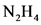is hydrazine.

Thehas no double or triple bonds present in the molecule.

The Lewis structure ofis:

Part 1

The Lewis structure of is:

Explanation | Common mistakes | Hint for next step

In, the total valence electrons are 14 electrons. Nitrogen has 5 valence electrons. Hydrogen needs only 2 electrons to complete its octet. Thus, the hydrogen forms bond single bonds between the two nitrogen. The two nitrogen forms the single bond with each other. Thus, there is one lone pair of electron on each nitrogen.

In the Lewis structures, the bond is represented by a straight line and not by dots.

Draw the Lewis structure for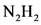.

### Step 2 of 3

(2)

The name ofis Diazene.

The structure of the compound is:

Part 2

The structure of the compound is:

Explanation | Common mistakes | Hint for next step

The nitrogen has 5 valance electrons. The two hydrogens present in the molecule form the single bond with each nitrogen. The octet of the nitrogen is completed by forming the double bond between both nitrogens. The one lone pair of electrons is present on each nitrogen.

There is no single bond between both nitrogens of the compound.

Draw the Lewis structure for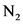.

### Step 3 of 3

(3)

Theis the nitrogen gas is inert in nature.

The Lewis structure of the compound is:

Part 3

The Lewis structure of the compound is:

Explanation | Common mistakes

The nitrogen atom has 5 valence electrons. The octet of the nitrogen is completed by forming the triple bond between both nitrogen atoms. The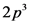electrons of both the nitrogen are used up to form the triple bond. The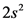electrons of the nitrogen are present as the lone pair of electrons.

In the Lewis structures, the bond is represented by a straight line and not by dots.

Part 1

The Lewis structure of is:

Part 2

The structure of the compound is:

Part 3

The Lewis structure of the compound is:

Part 1

The Lewis structure of is:

Part 2

The structure of the compound is:

Part 3

The Lewis structure of the compound is:

H-N-N-H # # N=N :N= N: H-N-N-H # # H-N-N-H # # HN-N&H We were unable to transcribe this image